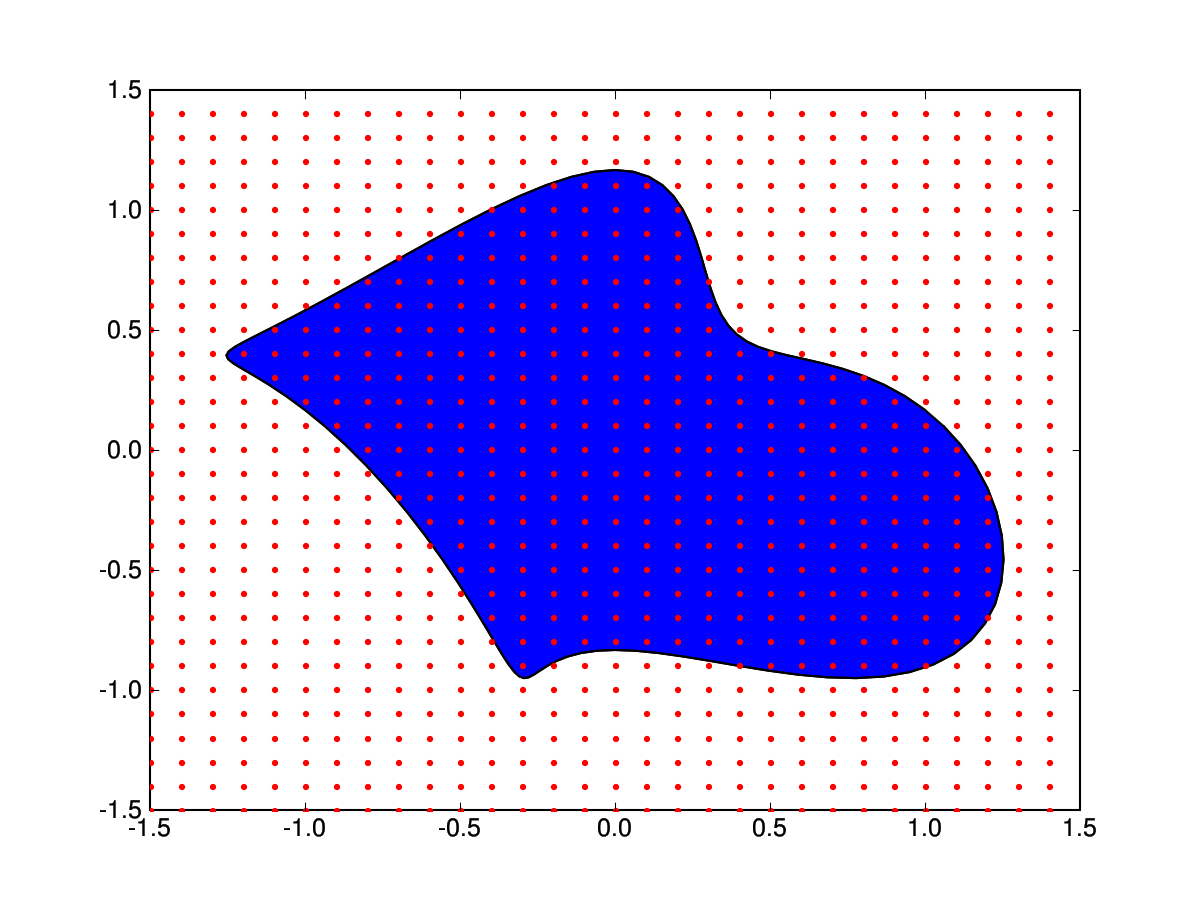# meshgrid

Hi all,

Is there a simple way to reject the points outside the parametrized curve ?
I mean I would like to use meshgrid for "arbitrary" shaped domains.

Any idea would be appreciated.

Nils

contour.py (326 Bytes)

Nils Wagner wrote:

Hi all,

Is there a simple way to reject the points outside the parametrized curve ?
I mean I would like to use meshgrid for "arbitrary" shaped domains.

Any idea would be appreciated.

Nils

------------------------------------------------------------------------

from scipy import *
from pylab import plot, show, meshgrid
N = 100
phi = linspace(0,2*pi,N,endpoint=False)
p = zeros(N, Complex)
q = zeros(N, Complex)
p.real = cos(phi)-0.5*cos(phi)*sin(2*phi)
p.imag = sin(phi)+cos(4*phi)/6
x=arange(-1.5,1.5,0.1)
y=arange(-1.5,1.5,0.1)
X,Y = meshgrid(x,y)
plot(p.real,p.imag,X,Y,'r.')
show()

Sorry for replying to myself but just now I have found the fill command.
Is it somehow possible to extract coordinates from the blue domain (image.png) ?

from scipy import *
from pylab import plot, show, meshgrid, fill
N = 100
phi = linspace(0,2*pi,N,endpoint=False)
p = zeros(N, Complex)
q = zeros(N, Complex)
p.real = cos(phi)-0.5*cos(phi)*sin(2*phi)
p.imag = sin(phi)+cos(4*phi)/6
x=arange(-1.5,1.5,0.1)
y=arange(-1.5,1.5,0.1)
X,Y = meshgrid(x,y)
plot(p.real,p.imag,'k-',X,Y,'r.')
q = fill(p.real,p.imag)
show()

NilsHi Nils,

I've found an algorithm for checking whether a point is inside a 2D polygon:

Please see the attached script where I've extended your example with the
corresponding python code
to find and draw points inside the domain.

There is a slow version and faster one using arrays. The slow version
works, but the "faster" one doesn't.
Does anyone see my mistake?

Michael.

blue-domain.py (2.58 KB)

I was browsing around and came across this alternative to Michael's:
<http://mu.arete.cc/pcr/syntax/pointInPolygon/1/pointInPolygon.py>

Gary R.

Michael Roettger wrote:

···

Hi Nils,

I've found an algorithm for checking whether a point is inside a 2D polygon:

http://www.alienryderflex.com/polygon/
Please see the attached script where I've extended your example with the
corresponding python code
to find and draw points inside the domain.

There is a slow version and faster one using arrays. The slow version
works, but the "faster" one doesn't.
Does anyone see my mistake?

Michael.